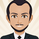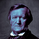3654浏览

The Adaptive Laguerre Filter was originally developed and described by John Ehlers in his paper `Time Warp – Without Space Travel`.

Thanks to @apozdnyakov for the sorting solution.

• Refactored
A list of Free indicators:
https://bit.ly/2S7EPuN

A list of Paid indicators:
https://bit.ly/33MA81f

Earn \$30:

Toss a coin to your witcher:
PayPal: https://www.paypal.me/alexeverget

## 评论novak.aleksey
@novak.aleksey, Hi. I did it 1 month ago. Sorry for the late response.Nice work! Thanks for sharing :)

After going through the code, I convinced myself of some changes... Can you verify?

1) Line 16: replace "length" with "medianLength"
2) Line 13: replace "i" with "(i-1)"
...if medianLength = 5, then ceil(medianLength/2) = 3. If line 13 iterates from 0 to 3, that's 4 iterations. Thus grabbing the 4th minimum value, not the 3rd (median of 5).

Here's my rendition of the adaptive part of the Laguerre filter:

length = 20
medianLength = 5
alpha = na
alf = na

error = abs(src - nz(alf))
range = highest(error, length) - lowest(error, length)
perc = range != 0 ? (error - lowest(error, length))/range : nz(alpha)
alpha := percentile_nearest_rank(perc, medianLength, 50.0) // Get value in the set, where 50% of all values are <= this value
//alpha := percentile_linear_interpolation(perc, medianLength, 50.0) // A variation on Ehler's definition

// ... continue with Laguerre filter

----------------------------------

Ironically, I like the behavior of your code, where the 4th value (instead of 3rd) is returned for alpha. This makes the filter more responsive, while still mitigating noise. In my code, if I set the percentile to 75.0 (instead of 50.0), I get the same results as your code.

Cheersbrobearbrobear
@brobear, @brobear, 1) Line 16: replace "length" with "medianLength"
2) Line 13: replace "i" with "(i-1)"
...if medianLength = 5, then ceil(medianLength/2) = 3. If line 13 iterates from 0 to 3, that's 4 iterations. Thus grabbing the 4th minimum value, not the 3rd (median of 5).
I can't find the corresponding code，can you post a complete script？ thank you！levith
@levith, look at the changelog - there were many updates. The current version is correctecletv
@ecletv, could you share a link to the volatility script you are using? Thanks.